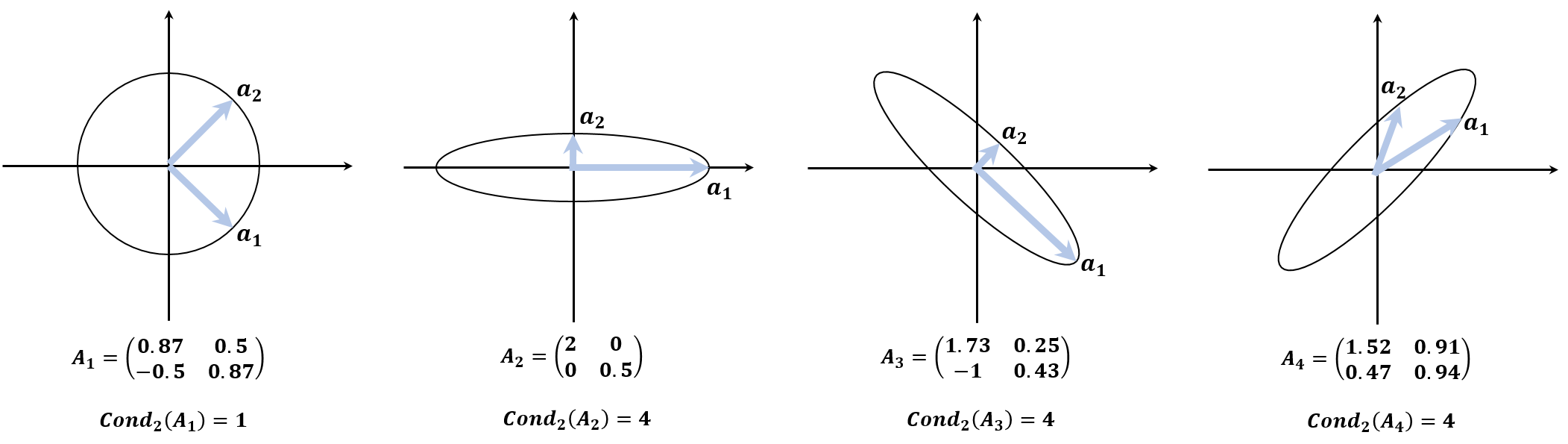# Condition Number

For a nonsingular square matrix $A$, its condition number is \begin{aligned} Cond(A) = \left\| A \right\| \left\| A^{-1} \right\| = \left( \max_{x \not = 0} \frac{\left\| Ax \right\|}{\left\| x \right\|} \right) \left( \min_{x \not = 0} \frac{\left\| Ax \right\|}{\left\| x \right\|} \right)^{-1} \end{aligned}

The condition number of a matrix denotes the ratio of the maximum relative stretching to the maximum relative shrinking to any nonzero vectors. In other words, it measures the amount of distortion of the unit sphere. Therefore, the condition number is not large when $A$ is a scaling matrix which stretches evenly to all the axes. When $A$ stretches espacially more to some axes, the condition number is larger. By convention, $Cond(A) = \infty$ if $A$ is singular.

• Euclidean condition number is
\begin{aligned} \left\| A \right\|_2 \left\| A^{-1} \right\|_2 = \sqrt{\frac{\lambda_{\max}(A^t A)}{\lambda_{\min}(A^t A)}} \end{aligned}

where $\lambda_{\max}(X)$ and $\lambda_{\min}(X)$ are the maximum and minimum eigenvalues of $X$. If $A$ symmetric, then $A^t Ax = A(\lambda x) = \lambda^2 x$ where $x$ and $\lambda$ are the eigenvector and eigenvalue of $A$, so \begin{aligned} \left\| A \right\|_2 \left\| A^{-1} \right\|_2 = \sqrt{\frac{\lambda_{\max}(A^t A)}{\lambda_{\min}(A^t A)}} = \left\vert \frac{\lambda_{\max}(A)}{\lambda_{\min}(A)} \right\vert \end{aligned}

The large the condition number is, the large the amount of distortion of the unit sphere is.• $Cond(A) \ge 1$ and $Cond(I) = 1$.
• $Cond(\gamma A) = Cond(A)$ where $\gamma \in \mathbb{R}$.
• $Cond(D) = \frac{\max{\vert d_i \vert}}{\min{\vert d_i \vert}}$ where $D$ is a diagonal matrix and $d_i$ is the $i$-th element of $D$.
• The condition number $A$ tells us about how close to singularity $A$ is. On the other hand, $\det A$ is not a good measure. When $A$ is singular, $\det A = 0$, but it does not mean that $A$ is close to singularity. For example, $\det \alpha I_n = \alpha^n$ for $\alpha \in \mathbb{R}$. For $\vert \alpha \vert < 1$, however, the determinant is close to zero although $I_n$ is perfectly well-conditioned.
• When estimating the condition number, the hardest part would be calculating $\left\| A^{-1} \right\|$. Suppose that $Az = y$ and $A$ is nonsingular. Then $\left\| z \right\| = \left\| A^{-1} y \right\| \le \left\| A^{-1} \right\| \left\| y \right\|$, so $\frac{\left\| z \right\|}{\left\| y \right\|} \le \left\| A^{-1} \right\|$. If a good $y$ is selected which makes $\frac{\left\| z \right\|}{\left\| y \right\|}$ large enough, it can approximate to $\left\| A^{-1} \right\|$. First of all, it can be found by random. Second, it can be solved by $A^t y = c$ where $c$ is a vector whose elements are $1$ or $-1$ so that $c$ makes $y$ as large as possible. Finally, it can be $\left\vert \frac{\lambda_{\max}(A)}{\lambda_{\min}(A)} \right\vert$ in case of estimating Euclidean condition number.

## Reference

 Michael T. Heath, Scientific Computing: An Introductory Survey. 2nd Edition, McGraw-Hill Higher Education.